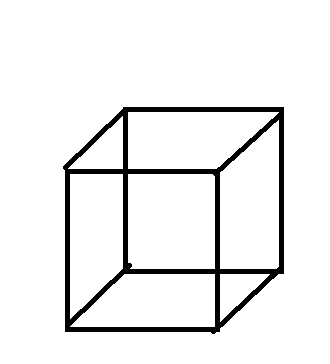Courses
Courses for Kids
Free study material
Free LIVE classes
MoreLIVE
Join Vedantu’s FREE Mastercalss

# The length of an edge of a cube is l. Find the formula for the sum of lengths of all the edges of the cube.Verified
359.4k+ views
Hint: In order to solve this problem, firstly draw the diagram of the cube and count the number of edges in it. Doing this will solve your problem and give you the right formula.The above diagram is of cube of edge length $l$.
So, sum of length of edges =12$l$
So, the sum of all of the lengths of the cube of edge length $l$ is 12$l$.